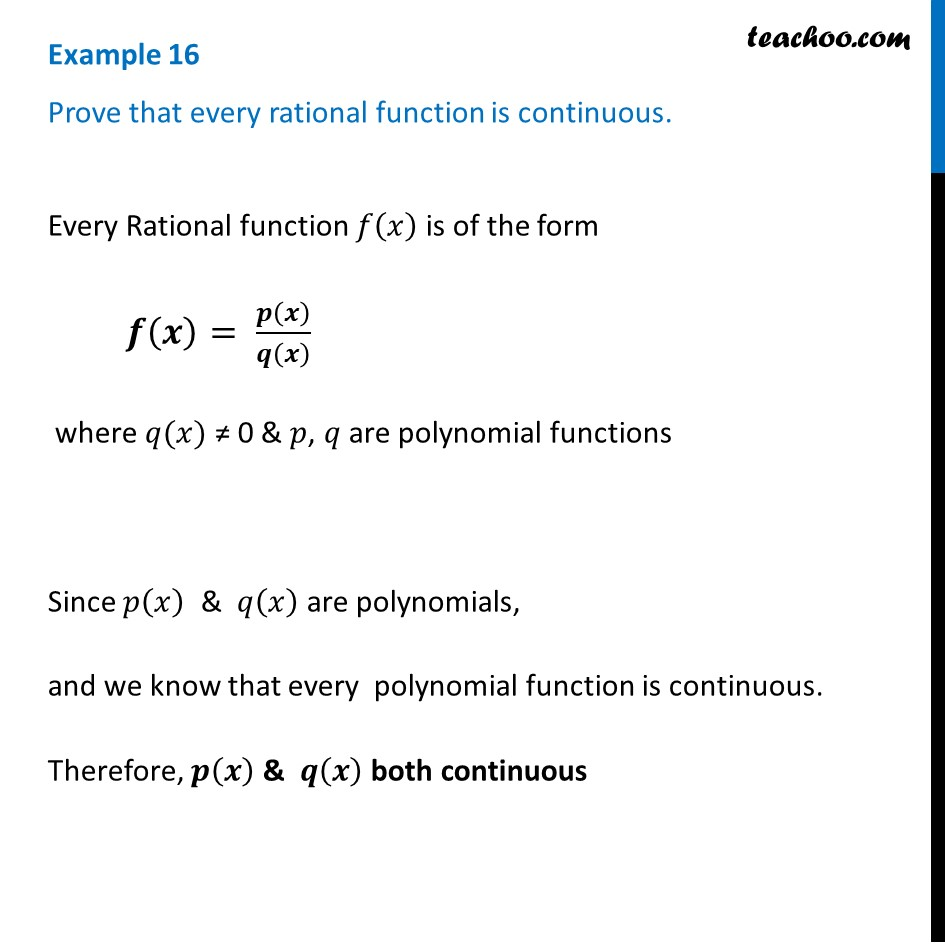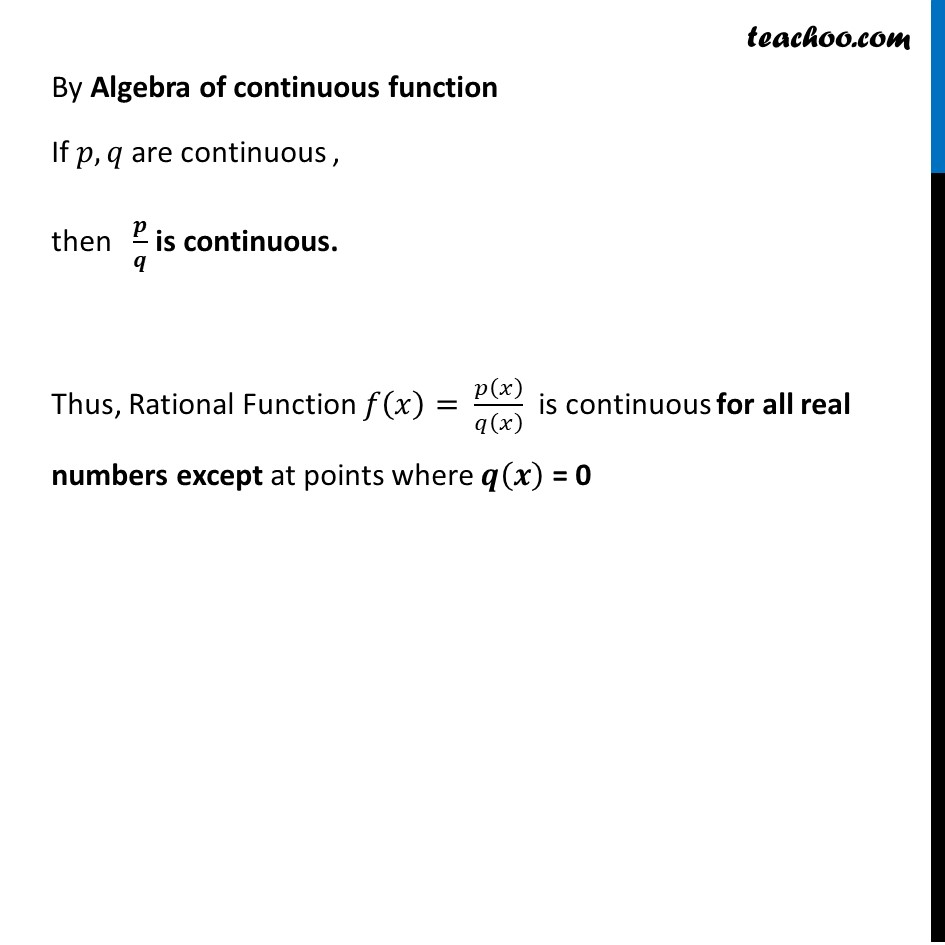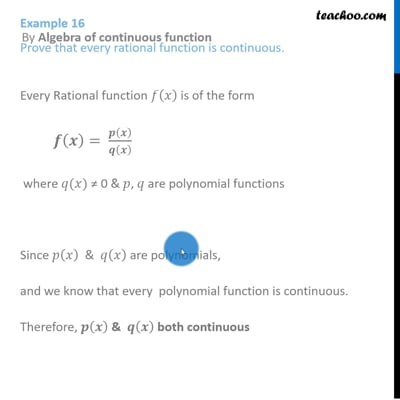Checking continuity at any point

Chapter 5 Class 12 Continuity and Differentiability
Concept wiseThis video is only available for Teachoo black users

Introducing your new favourite teacher - Teachoo Black, at only ₹83 per month

### Transcript

Example 16 Prove that every rational function is continuous.Every Rational function 𝑓(𝑥) is of the form 𝒇(𝒙)= 𝒑(𝒙)/𝒒(𝒙) where 𝑞(𝑥) ≠ 0 & 𝑝, 𝑞 are polynomial functions Since 𝑝(𝑥) & 𝑞(𝑥) are polynomials, and we know that every polynomial function is continuous. Therefore, 𝒑(𝒙) & 𝒒(𝒙) both continuous By Algebra of continuous function If 𝑝, 𝑞 are continuous , then 𝒑/𝒒 is continuous. Thus, Rational Function 𝑓(𝑥)= 𝑝(𝑥)/𝑞(𝑥) is continuous for all real numbers except at points where 𝒒(𝒙) = 0